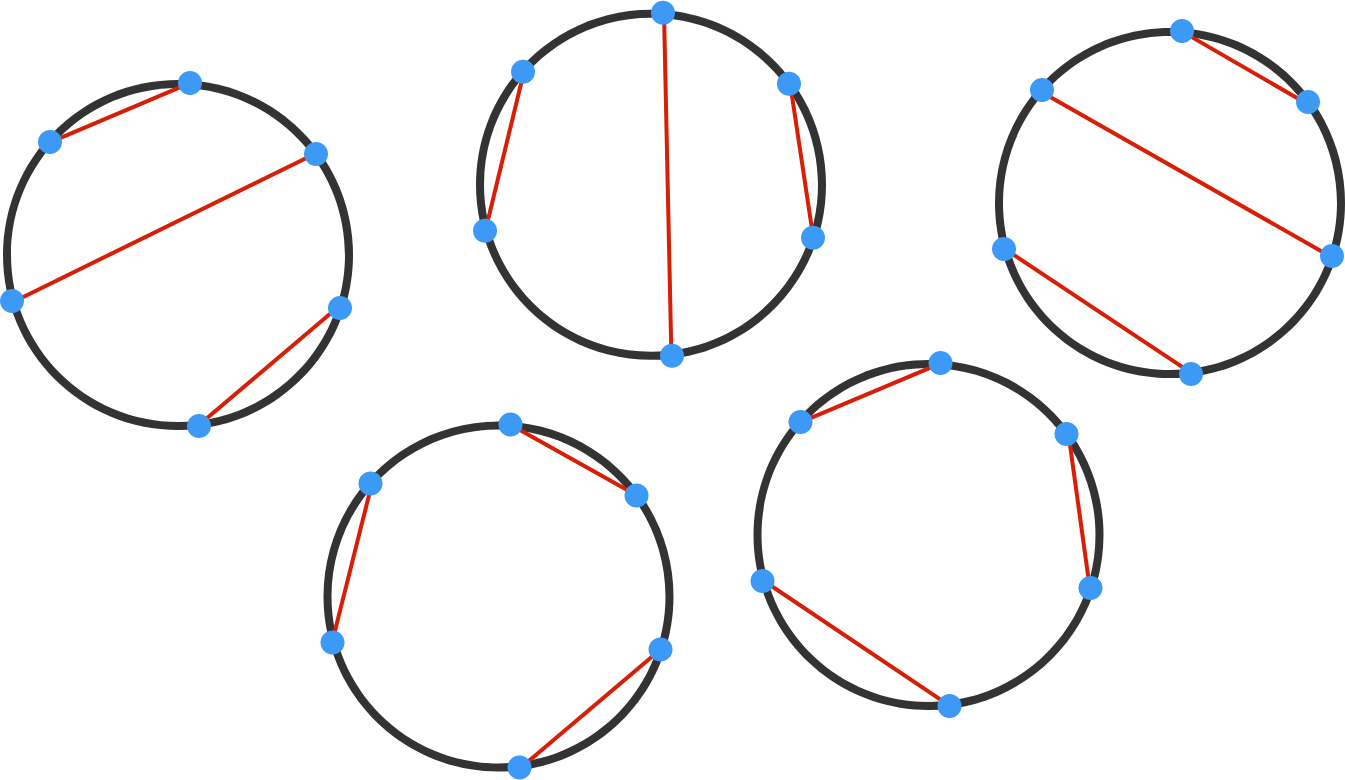# Nonintersecting chords of a circle

Probability Level 3

We label 10 points on the circumference of a circle as $P_1, P_2, \ldots, P_{10}.$ Find the number of ways to connect the points in pairs with non-intersecting chords, with no points left unconnected.


Hint: When the number of points is 6, the number of ways is 5, as shown below.×